# RankType Enum

Lists rank types.

Namespace: DevExpress.XtraPivotGrid

Assembly: DevExpress.PivotGrid.v20.2.Core.dll

## Declaration

``````[ResourceFinder(typeof(ResFinder), "PropertyNamesRes")]
public enum RankType``````

## Members

Name Description Example
`Competition`

Equal values receive the same ranking number, and a gap occurs in the ranking numbers.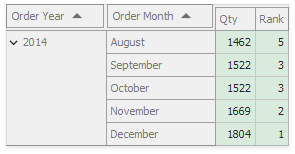`Dense`

Equal values receive the same ranking number, and the next item receives the following ranking number. No gap is left in ranking numbers.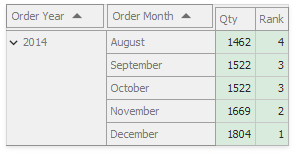`Unique`

Equal values receive distinct ordinal ranking numbers according to the order in which the values follow each other.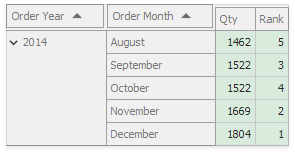`Modified`

Equal values receive the same ranking number, and a gap occurs in the ranking numbers before the equal-ranking items.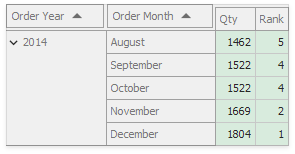`Percentile`

The rank is the percentage of values that are equal to or higher (if the order is descending), or equal to or lower (if the order is ascending) than the current value.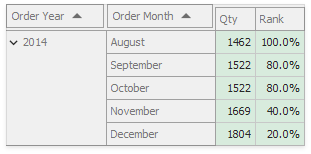## Related API Members

The following properties accept/return RankType values: